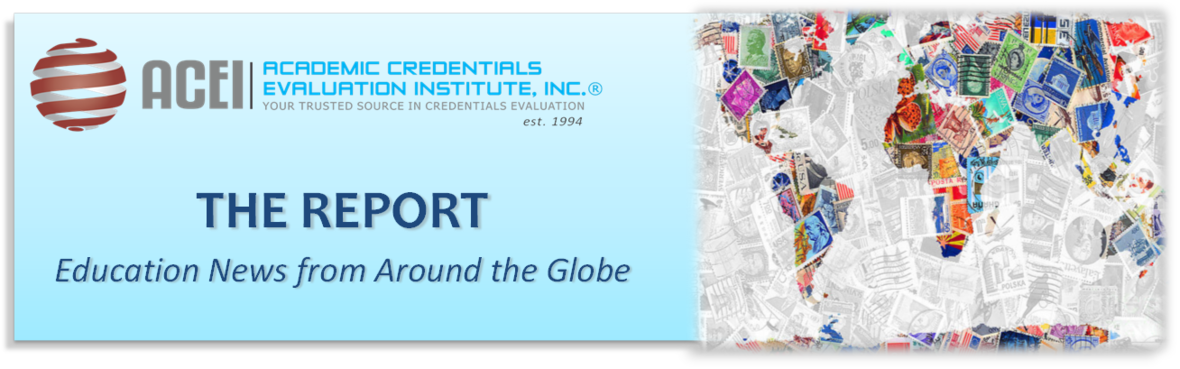# November 2019 - Beverly Hills, CA

ACADEMIC CREDENTIALS EVALUATION INSTITUTE, INC. (ACEI)
Is incorporated in the state of California (1994), and is dedicated to the advancement of international academic exchange and understanding through the dissemination of information on international academic credentials.

 table div table+table+table div table{width:100%;padding:0}table div table+table+table div table img{width:96.23%;padding:0;float:none}table div table+table+table div table td{width:100%;padding:0 1.88% 18px}/* styles */table div table+table+table+table+table div table{width:100%;padding:0}table div table+table+table+table+table div table img{width:96.23%;padding:0;float:none}table div table+table+table+table+table div table td{width:100%;padding:0 1.88% 18px}/* styles */table div table+table+table+table+table+table+table div table{width:100%;padding:0}table div table+table+table+table+table+table+table div table table{padding:0;float:left!important;width:37.924%!important}table div table+table+table+table+table+table+table div table table+table td,table div table+table+table+table+table+table+table div table td{padding-left:0;padding-right:0}table div table+table+table+table+table+table+table div table table td{padding-left:0;padding-right:20px}table div table+table+table+table+table+table+table div table table+table{float:left!important;width:62.076%!important}/* styles */
 table div table+table+table+table+table+table+table+table+table div table{width:100%;padding:0}table div table+table+table+table+table+table+table+table+table div table img{width:96.23%;padding:0;float:none}table div table+table+table+table+table+table+table+table+table div table td{width:100%;padding:0 1.88% 18px}/* styles */# News Around the world# MP hired imposters to take her exams

A Bangladeshi politician has been expelled from university after allegedly hiring as many as eight lookalikes to take her place in exams. To read more, click here.

 table div table+table+table+table+table+table+table+table+table+table+table+table+table+table div table{width:100%;padding:0}table div table+table+table+table+table+table+table+table+table+table+table+table+table+table div table img{width:96.23%;padding:0;float:none}table div table+table+table+table+table+table+table+table+table+table+table+table+table+table div table td{width:100%;padding:0 1.88% 18px}/* styles */# Fighting Fake News the Canadian Way

The need for real journalism has never been greater, with governments abusing the term to pass anti-press laws, presidents sidestepping accountability by dismissing reports as “fake news”, and with authoritarian leaders weaponizing these stories to cement power. Concordia University’s Institute for Investigative Journalism is stepping in to change this landscape for the better. To read more, click here

 table div table+table+table+table+table+table+table+table+table+table+table+table+table+table+table+table div table{width:100%;padding:0}table div table+table+table+table+table+table+table+table+table+table+table+table+table+table+table+table div table img{width:96.23%;padding:0;float:none}table div table+table+table+table+table+table+table+table+table+table+table+table+table+table+table+table div table td{width:100%;padding:0 1.88% 18px}/* styles */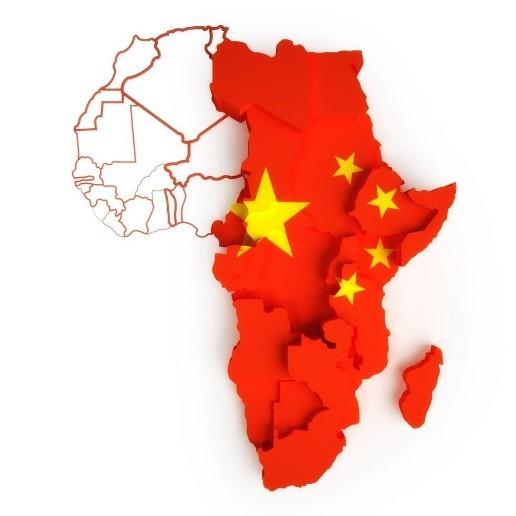# Teaching Local African Language

One of China’s oldest universities, the Beijing Foreign Studies University (BFSU), announced the introduction last month of a course in Kinyarwanda – one of Rwanda’s four official languages which is spoken by almost all of the native population and 12 million people in Rwanda, Eastern Democratic Republic of the Congo and adjacent parts of southern Uganda. To read more, click here.

 table div table+table+table+table+table+table+table+table+table+table+table+table+table+table+table+table+table+table div table{width:100%;padding:0}table div table+table+table+table+table+table+table+table+table+table+table+table+table+table+table+table+table+table div table img{width:96.23%;padding:0;float:none}table div table+table+table+table+table+table+table+table+table+table+table+table+table+table+table+table+table+table div table td{width:100%;padding:0 1.88% 18px}/* styles */# A New School to Train Spies

Germany’s intelligence agencies on Tuesday inaugurated a joint training center in the heart of Berlin, a city that was dubbed the ‘capital of spies’ during the Cold War and that to this day remains a hot spot of espionage. To read more, click here.

 table div table+table+table+table+table+table+table+table+table+table+table+table+table+table+table+table+table+table+table+table div table{width:100%;padding:0}table div table+table+table+table+table+table+table+table+table+table+table+table+table+table+table+table+table+table+table+table div table img{width:96.23%;padding:0;float:none}table div table+table+table+table+table+table+table+table+table+table+table+table+table+table+table+table+table+table+table+table div table td{width:100%;padding:0 1.88% 18px}/* styles */# New Vocational Bachelor & Master Degrees:

A law introducing new degree titles: "Bachelor Professional" & "Master Professional" for vocational further education has been approved by the German federal parliament, despite strong criticism by higher education heads, who fear that it could damage the Bologna higher education reform process. To read more, click here.

 table div table+table+table+table+table+table+table+table+table+table+table+table+table+table+table+table+table+table+table+table+table+table+table div table{width:100%;padding:0}table div table+table+table+table+table+table+table+table+table+table+table+table+table+table+table+table+table+table+table+table+table+table+table div table img{width:96.23%;padding:0;float:none}table div table+table+table+table+table+table+table+table+table+table+table+table+table+table+table+table+table+table+table+table+table+table+table div table td{width:100%;padding:0 1.88% 18px}/* styles */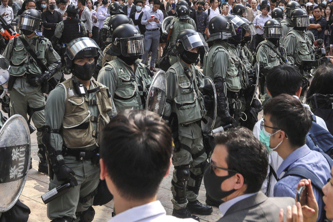Photo credit: Nora Tam

# Protests are Impacting Education

The anti-government protests continue in Hong Kong. The protesters–many young high school and university students–have dug in at several university campuses across Hong Kong. For quick facts, click here. For a more detailed read, click here.

 table div table+table+table+table+table+table+table+table+table+table+table+table+table+table+table+table+table+table+table+table+table+table+table+table+table+table div table{width:100%;padding:0}table div table+table+table+table+table+table+table+table+table+table+table+table+table+table+table+table+table+table+table+table+table+table+table+table+table+table div table img{width:96.23%;padding:0;float:none}table div table+table+table+table+table+table+table+table+table+table+table+table+table+table+table+table+table+table+table+table+table+table+table+table+table+table div table td{width:100%;padding:0 1.88% 18px}/* styles */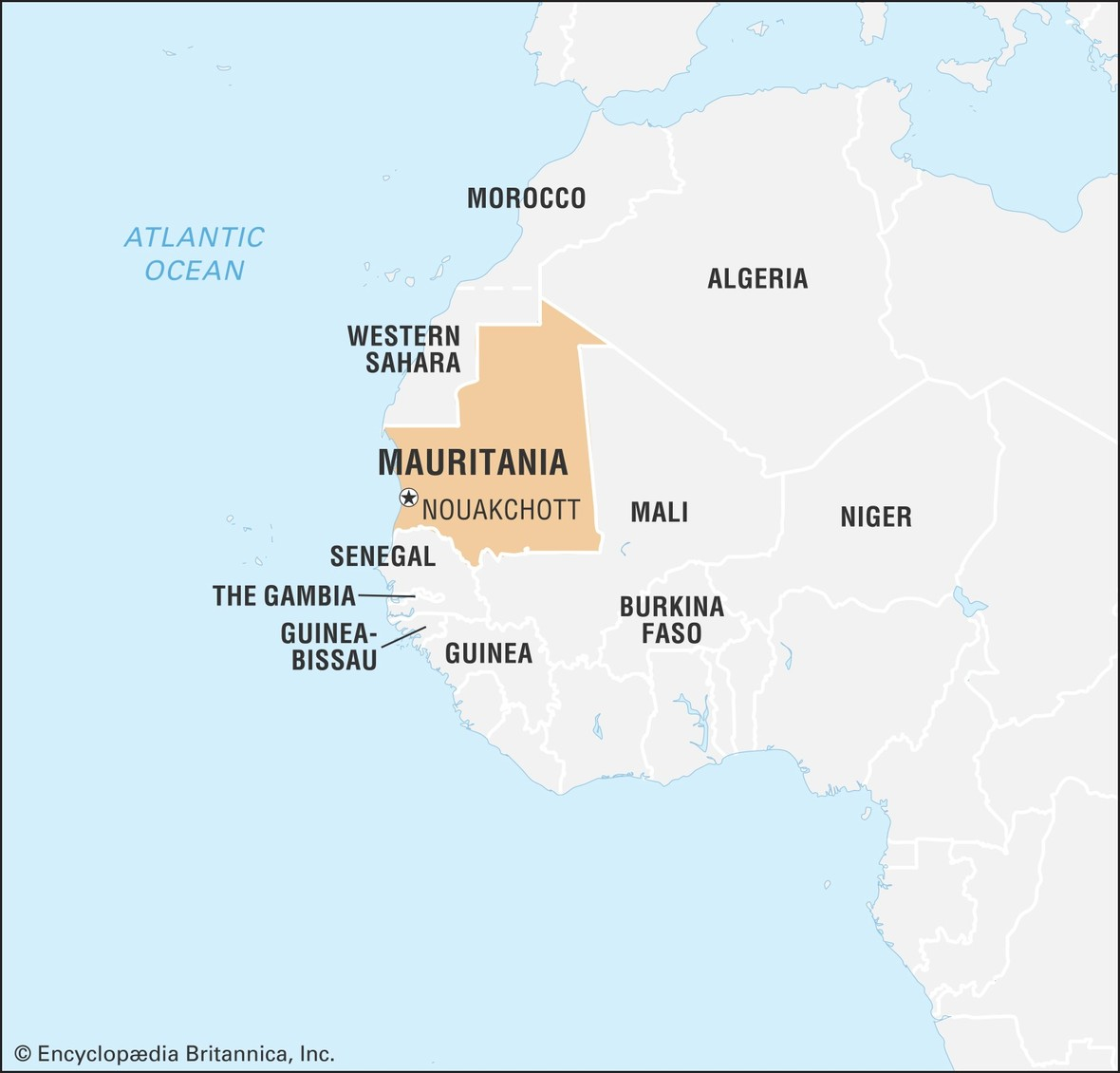# University Entrance Age Limit Lifted

The Mauritanian government has removed the age limit of 25 years for university entry after sustained protest action by students over the past weeks. To read more, click here.

 table div table+table+table+table+table+table+table+table+table+table+table+table+table+table+table+table+table+table+table+table+table+table+table+table+table+table+table+table div table{width:100%;padding:0}table div table+table+table+table+table+table+table+table+table+table+table+table+table+table+table+table+table+table+table+table+table+table+table+table+table+table+table+table div table img{width:96.23%;padding:0;float:none}table div table+table+table+table+table+table+table+table+table+table+table+table+table+table+table+table+table+table+table+table+table+table+table+table+table+table+table+table div table td{width:100%;padding:0 1.88% 18px}/* styles */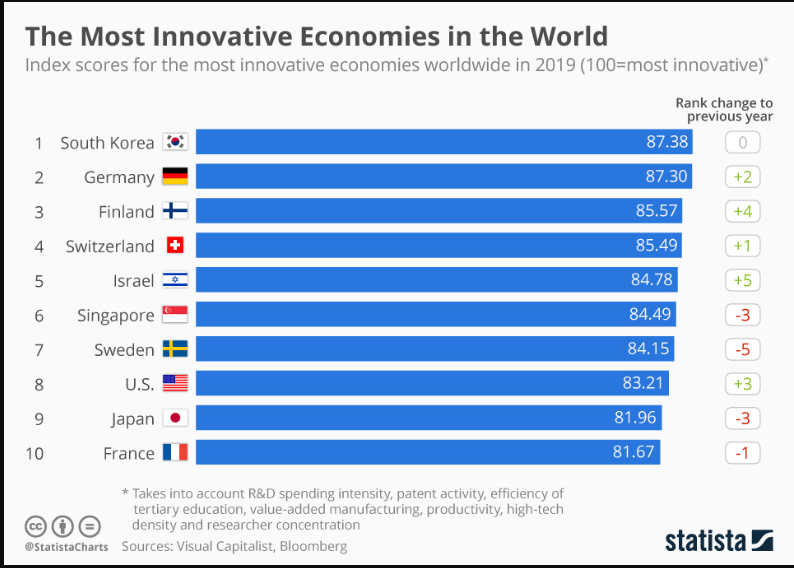# Most Innovative Economy in the World

South Korea has been named the world's most innovative economy for the second year running. 'Innovation' takes into account R&D, efficient education, technology advancements and manufacturing skills. To read more, click here.

 table div table+table+table+table+table+table+table+table+table+table+table+table+table+table+table+table+table+table+table+table+table+table+table+table+table+table+table+table+table+table+table div table{width:100%;padding:0}table div table+table+table+table+table+table+table+table+table+table+table+table+table+table+table+table+table+table+table+table+table+table+table+table+table+table+table+table+table+table+table div table img{width:96.23%;padding:0;float:none}table div table+table+table+table+table+table+table+table+table+table+table+table+table+table+table+table+table+table+table+table+table+table+table+table+table+table+table+table+table+table+table div table td{width:100%;padding:0 1.88% 18px}/* styles */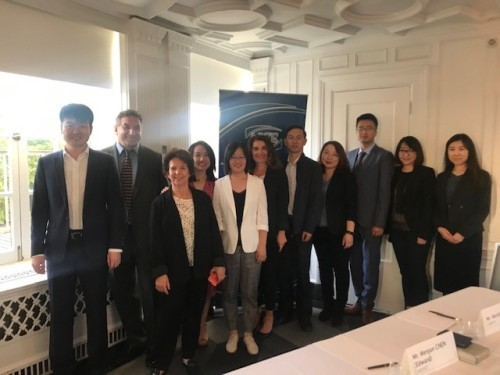# Sharing Expertise on International Credential Evaluations

On October 18, 2019, the Academic Credentials Evaluation Institute (ACEI) had the pleasure of hosting representatives from the Chinese Service Center for Scholarly Exchange (CSCSE) and China Higher Education Student Information and Career Center (CHESICC) and colleagues from the American Education Research Corporation (AERC). The purpose of the meeting was to learn more about our respective organizations and discuss the role of the US Department of Education and the Association of International Credential Evaluators (AICE). To read more, click here.

 table div table+table+table+table+table+table+table+table+table+table+table+table+table+table+table+table+table+table+table+table+table+table+table+table+table+table+table+table+table+table+table+table+table+table div table{width:100%;padding:0}table div table+table+table+table+table+table+table+table+table+table+table+table+table+table+table+table+table+table+table+table+table+table+table+table+table+table+table+table+table+table+table+table+table+table div table img{width:96.23%;padding:0;float:none}table div table+table+table+table+table+table+table+table+table+table+table+table+table+table+table+table+table+table+table+table+table+table+table+table+table+table+table+table+table+table+table+table+table+table div table td{width:100%;padding:0 1.88% 18px}/* styles */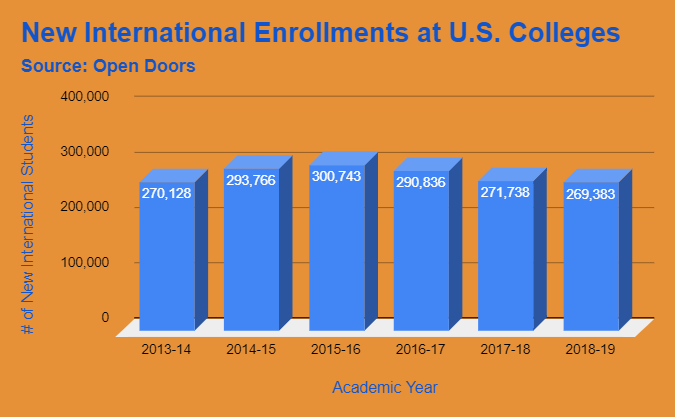# International Student Enrollments are Down

According to new data from IIE’s Open Doors, the number of international students enrolled at U.S. institutions of higher education dropped in academic year 2018-2019. To read more, click here. And, for the impact this decline is having on U.S. economy, click here.

 table div table+table+table+table+table+table+table+table+table+table+table+table+table+table+table+table+table+table+table+table+table+table+table+table+table+table+table+table+table+table+table+table+table+table+table+table+table div table{width:100%;padding:0}table div table+table+table+table+table+table+table+table+table+table+table+table+table+table+table+table+table+table+table+table+table+table+table+table+table+table+table+table+table+table+table+table+table+table+table+table+table div table img{width:96.23%;padding:0;float:none}table div table+table+table+table+table+table+table+table+table+table+table+table+table+table+table+table+table+table+table+table+table+table+table+table+table+table+table+table+table+table+table+table+table+table+table+table+table div table td{width:100%;padding:0 1.88% 18px}/* styles */# Upcoming Events## Upcoming Webinar:

So you think you're diverse? Examining your Institution's Diversity and Inclusion practice.
Free Webinar
Wednesday, December 11, 2019 10 AM - 11 AM PST

## Upcoming Events 2019:

2019 AIRC Annual Conference
ACEI will be attending and exhibiting at AIRC
December 11-14, 2019, Miami, FL

AICE 2020 Symposium
If evaluating professional qualifications in law and medicine from institutions outside the U.S. are conundrum for you, be sure to register for AICE’s 2020 Symposium on April 4th in New Orleans, LA. REGISTER NOW! For more information, please click here.

 table div table+table+table+table+table+table+table+table+table+table+table+table+table+table+table+table+table+table+table+table+table+table+table+table+table+table+table+table+table+table+table+table+table+table+table+table+table+table+table+table+table+table+table+table div table{width:100%;padding:0}table div table+table+table+table+table+table+table+table+table+table+table+table+table+table+table+table+table+table+table+table+table+table+table+table+table+table+table+table+table+table+table+table+table+table+table+table+table+table+table+table+table+table+table+table div table img{width:96.23%;padding:0;float:none}table div table+table+table+table+table+table+table+table+table+table+table+table+table+table+table+table+table+table+table+table+table+table+table+table+table+table+table+table+table+table+table+table+table+table+table+table+table+table+table+table+table+table+table+table div table td{width:100%;padding:0 1.88% 18px}/* styles */table.module-45{width:84.72%;padding:0}table div table+table+table+table+table+table+table+table+table+table+table+table+table+table+table+table+table+table+table+table+table+table+table+table+table+table+table+table+table+table+table+table+table+table+table+table+table+table+table+table+table+table+table+table+table+table div table{width:84.72%;float:none;margin-left:auto;margin-right:auto;padding:0}table div table+table+table+table+table+table+table+table+table+table+table+table+table+table+table+table+table+table+table+table+table+table+table+table+table+table+table+table+table+table+table+table+table+table+table+table+table+table+table+table+table+table+table+table+table+table div table a{border:0 none;text-decoration:none}table div table+table+table+table+table+table+table+table+table+table+table+table+table+table+table+table+table+table+table+table+table+table+table+table+table+table+table+table+table+table+table+table+table+table+table+table+table+table+table+table+table+table+table+table+table+table div table img{width:100%!important;border:0 none;text-decoration:none}table div table+table+table+table+table+table+table+table+table+table+table+table+table+table+table+table+table+table+table+table+table+table+table+table+table+table+table+table+table+table+table+table+table+table+table+table+table+table+table+table+table+table+table+table+table+table div table td{width:100%;padding:0}/* styles */

# Digital Delivery of Evaluation Reports: SecurePathway©:

ACEI is a proud signatory of the Groningen Declaration Network which “seeks common ground in best serving the academic and professional mobility needs of citizens worldwide by bringing together key stakeholders in the Digital Student Data Ecosystem.” For the past two years, ACEI has committed itself to this shared mission through the introduction of SecurePathway©, an ACEI proprietary digital platform for the secure delivery of official evaluation reports and supporting educational credentials. For information on ACEI’s SecurePathway©.

Signing up with ACEI’s SecurePathway©, is free and does not cost the institutions any fees. To receive ACEI expert reports and certified academic credentials via SecurePathway©, complete this form.

*SecurePathway Registration Form*/* styles */ ACEI’s Advisory Services provide institutions and organizations looking at expanding their international student recruitment with customized solutions to help overcome enrollment challenges, uncover opportunities, and devise an innovative and holistic approach to international enrollment. For more information on ACEI’s Advisory Services and how we can assist you, please contact Yolinisse Moreno, Director of Programs & Communications at ymoreno@acei-global.org.
 table div table+table+table+table+table+table+table+table+table+table+table+table+table+table+table+table+table+table+table+table+table+table+table+table+table+table+table+table+table+table+table+table+table+table+table+table+table+table+table+table+table+table+table+table+table+table+table+table+table+table div table{width:100%;padding:0}table div table+table+table+table+table+table+table+table+table+table+table+table+table+table+table+table+table+table+table+table+table+table+table+table+table+table+table+table+table+table+table+table+table+table+table+table+table+table+table+table+table+table+table+table+table+table+table+table+table+table div table img{width:96.23%;padding:0;float:none}table div table+table+table+table+table+table+table+table+table+table+table+table+table+table+table+table+table+table+table+table+table+table+table+table+table+table+table+table+table+table+table+table+table+table+table+table+table+table+table+table+table+table+table+table+table+table+table+table+table+table div table td{width:100%;padding:0 1.88% 18px}/* styles *//* styles */ ACEI offers a comprehensive range of professional services that include: international credential evaluations; translations; consulting; and training. For more information on ACEI, please visit www.acei-global.org or contact: Tel: 1-310-275-3530; Email: acei@acei-global.org. ACEI was founded and incorporated in 1994 in the State of California and is a Charter and Endorsed Member of the Association of International Credential Evaluators (AICE).
 Like   Tweet   Pin   +1   in